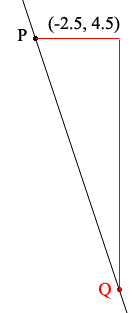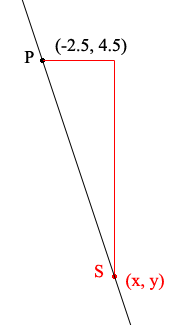SEARCH HOMEMath Central Quandaries & QueriesQuestion from Sava, a student: I have to write an equation and sketch a graph for the line that meets the given conditions: A line with slope -15/5 that passes through the point (-2.5,4.5)Hi Sava,

Since -15/5 = -3 you line has a slope of -3. This is negative so if you are walking along the line in the coordinate plane going from left to right you are going downhill.In fact you know that if you move from the point P (-2.5, 4.5) and go horizontally one unit to the right and vertically 3 units downward you arrive at a point Q on the line. What are the coordinates of Q? Plot P and Q accurately on graph paper and join them to obtain a sketch of the graph of the line.

To find the equation of the line let S be a point on the line with coordinates (x, y).What is the horizontal distance between P and S? (This is the run.) What is the vertical distance between P and S? (This is the rise.) Since I took S to the right of P the horizontal distance is positive and the vertical distance is negative. The rise divided by the run is the slope which you know is -3 so if you form the equation

rise/run = -3

you will have the equation of the line.

Simplify this expression and then go through the process again but with S to the left of P. Simplify the equation you get in this case and verify the two equations are identical.

I hope this helps,
PennyMath Central is supported by the University of Regina and The Pacific Institute for the Mathematical Sciences.